Your data analysis solution

# Quantiles or percentiles computation in Excel

2017-10-20

This tutorial shows how to compute quantiles or percentiles associated to confidence intervals in Excel using the XLSTAT software.

## Quantiles and percentiles

XLSTAT has a complete tool to compute quantiles or percentiles, their associated confidence interval and graphical representations.

Quantiles are important statistical measures, they are simple to understand. The 0.5-quantile is the value such that half of the sample is below and the other half is above. It is also called the median. A quantile is called a percentile when it is based on a 0-100 scale. The 0.95-quantile is equivalent to the 95-percentile and is such that 95 % of the sample is below its value and 5 % is above.

## Dataset to generate a quantile

An Excel sheet with both the data and results can be downloaded by clicking here.

The dataset has been obtained from [Lewis T. and Taylor L.R. (1967). Introduction to Experimental Ecology, New York: Academic Press, Inc]. It concerns 237 children, described by their gender and height in centimeters (1 cm = 0.4 inch).

## Setting up the computation of a specific quantile

After opening XLSTAT, select the XLSTAT / Description / Quantiles, or click on the corresponding button of the "Description" toolbar (see below).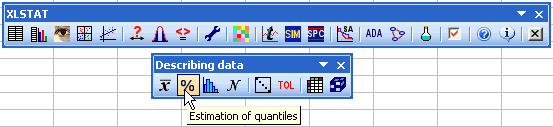Once you've clicked on the button, the Quantile dialog box will appear. Select the data on the Excel sheet.

In our case; the variable is the "Height". Data should be quantitative.

As the column header was selected for the variables, the Variable labels option needs to be activated.

We select the default method for estimation (weighted mean at x(Np)) and both type of confidence intervals with a 95 % confidence.

Details on statistical methods can be found in the help of XLSTAT.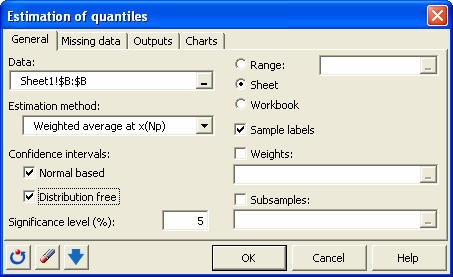In the charts tab, we select all charts and we are interested by the 67-percentile (two third of the children are smaller and one third is taller).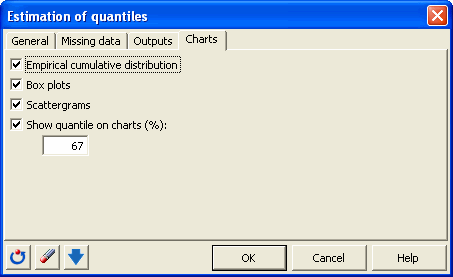The computations begin once you have clicked on OK. The results will then be displayed.

## Interpreting the results of a quantile generation

The first table displays some descriptive statistics on the height variable. The second table displays quantiles and their associated confidence interval for different commonly used values. For example, the median is 159.9 cm. The 95-percentile shows that 95 % of the children are smaller than 174.98 cm.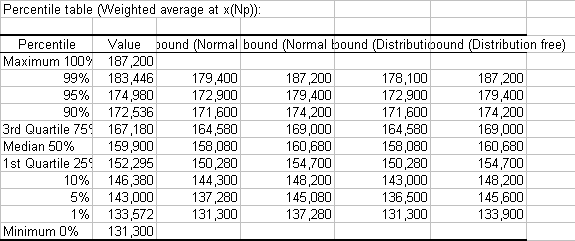The value of the 67-percentile is then displayed. Two third of the children are smaller than 164.58 cm.

The first chart (see below) allows us to visualize the empirical cumulative distribution function with the value of the 67-percentile.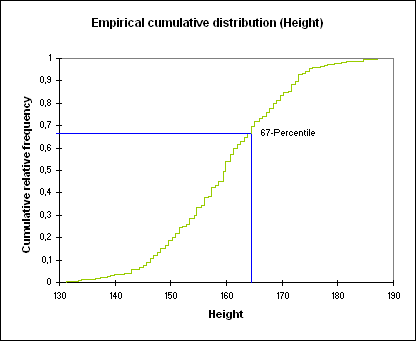The second and third charts are a box plot and the scattergram. The 67-percentile is displayed using a blue line.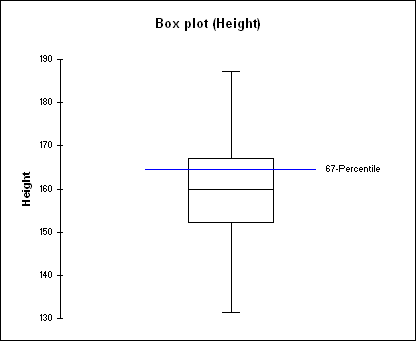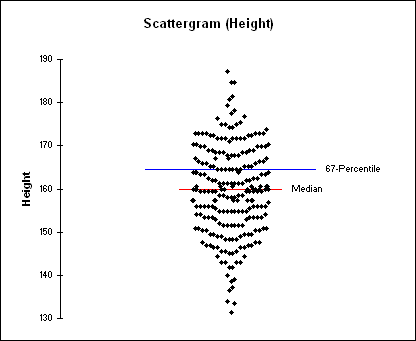You can also use sub-samples, for example gender can be used as a group variable. Weights associated to the observations can also be included.

### Contact Us

#### Contact our technical support team: support@xlstat.com

https://cdn.desk.com/
false
desk
Loading
seconds ago
a minute ago
minutes ago
an hour ago
hours ago
a day ago
days ago
about
false
Invalid characters found
/customer/portal/articles/autocomplete
9283